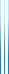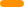# PublicationsPapers

## Twenty (short) questions

Yuval Dagan, Yuval Filmus, Ariel Gabizon and Shay Moran
Combinatorica

dyadic distribution is one in which all probabilities are negative powers of 2. If $\mu$ is a dyadic distribution on a finite domain, then Huffman’s algorithm produces a code whose average codeword length is $H(\mu)$. We can interpret this code as a decision tree.

A dyadic distribution can have many different optimal decision trees. We are interested in the following question: given $n$, what is the smallest list of queries that suffices to implement optimal decision trees for all dyadic distributions on $n$ elements?

We show that $1.25^{n+o(1)}$ queries suffice, and moreover, $1.25^{n-o(1)}$ queries are necessary for infinitely many $n$.

We also discuss how the number of queries scales as we allow slight deviations from optimality.

This paper is extracted from a longer STOC paper.

## BibTeX

@article{DFGM2019,
author = {Yuval Dagan and Yuval Filmus and Ariel Gabizon and Shay Moran},
title = {Twenty (short) questions},
journal = {Combinatorica},
volume = {39},
number = {3},
pages = {597--626},
year = {2019}
}copy to clipboard